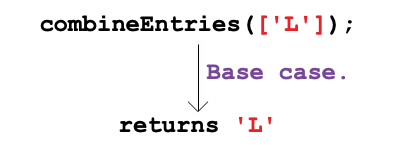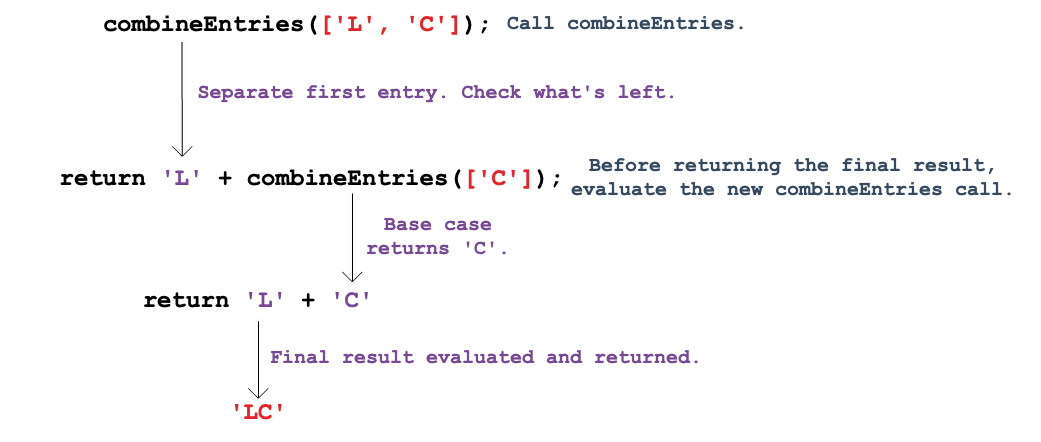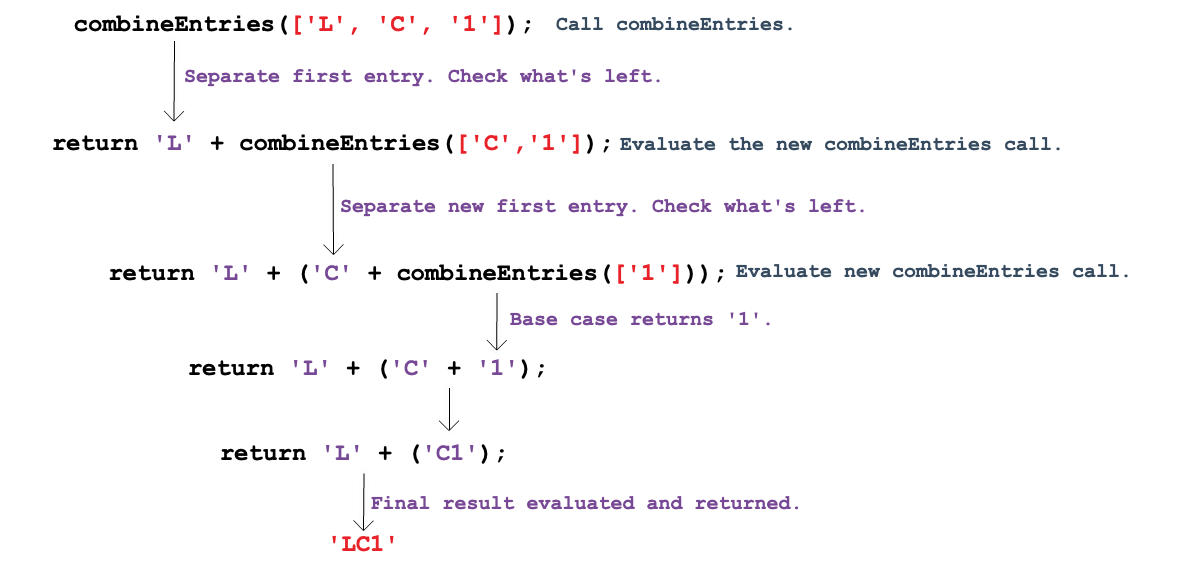# Making a Function Call Itself

Congratulations! Identifying the base case is often the trickiest part of building a recursive function.

We’ve made it this far with `combineEntries`:

``````function combineEntries(arrayName){
if (arrayName.length <= 1){
return arrayName;
} else {
//call combineEntries again
}
}
``````

Now we are ready to take the next step.

## A Visual Representation

To help visualize what happens during recursion, let’s start with the base case `['L']`:Nothing complicated here. `combineEntries` sees only one item in the array, so it returns `'L'`.

Now consider an array with two elements, `['L', 'C']`:In this case, `combineEntries` executes the `else` statement. We have no code for this yet, but we can still consider the logic:

1. `combineEntries` returns `'L'` and calls itself again using what is left inside the array (`['C']`).
2. When passed `['C']`, which is the base case, `combineEntries` returns `'C'`.
3. The strings `'L'` and `'C'` get combined and returned as the final result.

Next, consider an array with three elements `['L', 'C', '1']`:As before, `combineEntries` executes the `else` statement, and we can follow the logic:

1. `combineEntries` returns `'L'` and calls itself again using what is left inside the array (`['C', '1']`).
2. When passed `['C', '1']`, `combineEntries` returns `'C'` and calls itself again using what is left inside the array (`['1']`).
3. When passed `['1']`, which is the base case, `combineEntries` returns `'1'`.
4. The strings `'C'` and `'1'` get combined and returned.
5. The strings `'L'` and `'C1'` get combined and returned as the final result.

As we make the array longer, `combineEntries` calls itself more times. Each call evaluates a smaller and smaller section of the array until reaching the base case. This sets up a series of return events - each one selecting a single entry from the array. Rather than building `'LC101'` from left to right, recursion constructs the string starting with the base case and adding new characters to the front:

Value ReturnedDescription
`'1'`Base case. Returns the element from an array of length 1.
`'01'`Combines the first element from an array of length 2 with the base case value.
`'101'`Combines the first element from an array of length 3 with the two previous values.
`'C101'`Combines the first element from an array of length 4 with the three previous values.
`'LC101'`Combines the first element from an array of length 5 with the four previous values.

Recursive processes all follow this approach. Each call to the function reduces a problem into a slighly smaller piece. The reduction continues until reaching the simplest possible form—the base case. The base case is then solved, and this creates a starting point for completing all of the previous steps.

## A Function Calls Itself

So how do we code the `else` statement in `combineEntries`? Recall what needs to happen each time the statement runs:

1. Select the first element in the array,
2. Call `combineEntries` again with a smaller array.

Bracket notation takes care of part a: `arrayName`.

For part b, remember that the slice methoed returns selected entries from an array. To return everything BUT the first entry in `arr = ['L', 'C', '1', '0', '1']``, use ``arr.slice(1)`.

Let’s add the bracket notation and the `slice` method to our function:

``````function combineEntries(arrayName){
if (arrayName.length <= 1){
return arrayName;
} else {
return arrayName+combineEntries(arrayName.slice(1));
}
}
``````

Each time the `else` statement runs, it extracts the first element in the array with `arrayName`, then it calls itself with the remaining array elements (`arrayName.slice(1)`).

For `combineEntries(['L', 'C', '1', '0', '1']);`, the sequence would be:

StepDescription
1First call: Combine `'L'` with `combineEntries(['C', '1', '0', '1'])`.
2Second call: Combine `'C'` with `combineEntries(['1', '0', '1'])`.
3Third call: Combine `'1'` with `combineEntries(['0', '1'])`.
4Fourth call: Combine `'0'` with `combineEntries(['1'])`.
5Fifth call: Base case returns `'1'`.

To get the final result, proceed up the chain:

StepDescription
5Return `'1'` to the fourth call.
4Return `'01'` to the third call.
3Return `'101'` to the second call.
2Return `'C101'` to the first call.
1Return `'LC101'` as the final result.